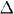# In moving out of a dormitory at the end of the semester, a s

• Alice7979

## Homework Statement

In moving out of a dormitory at the end of the semester, a student does 2.62 x 10^4 J of work. In the process, his internal energy decreases by 3.96 x 104 J. Determine each of the following quantities (including the algebraic sign): (a)W, (b)U, and (c)Q.

## Homework Equations

b) ∆U = Q-W

c) if the system does work its positive, if the system has work done on its negative

## The Attempt at a Solution

I got (a) right, it is 2.62 x 10^4 J

b)
-3.96E4 - (2.62E4) = -65800

c)
-3.96E4 but I'm not sure about if it is negative or positive

And does the algebraic sign just mean + or -?

#### Attachments

Alice7979 said:
And does the algebraic sign just mean + or -?
Yes (as opposed to giving just the absolute value).
Alice7979 said:
In the process, his internal energy decreases by 3.96 x 104 J.
This is the number you give for part c.
So the change in internal energy is Q?